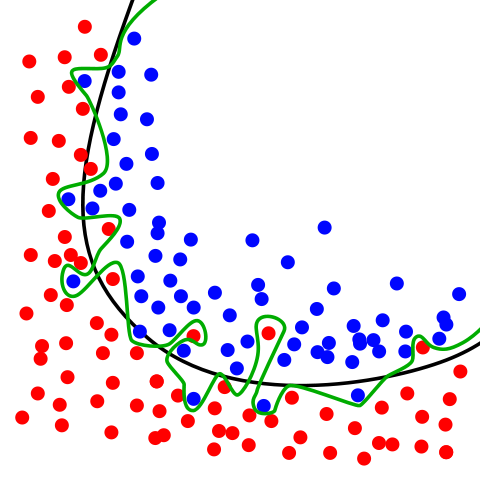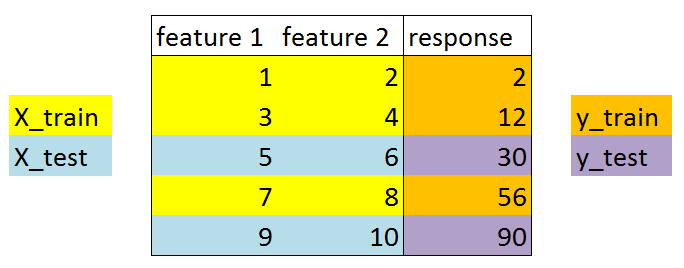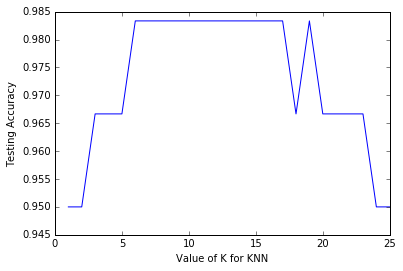Train a KNN classification model with scikit-learn
Train a KNN classification model with scikit-learn

## Topics¶

1. Evaluation procedure 1 - Train and test on the entire dataset
• a. Logistic regression
• b. KNN (k = 5)
• c. KNN (k = 1)
• d. Problems with training and testing on the same data
2. Evaluation procedure 2 - Train/test split
3. Making predictions on out-of-sample data
4. Downsides of train/test split
5. Resources

I would like to give full credits to the respective authors as these are my personal python notebooks taken from deep learning courses from Andrew Ng, Data School and Udemy :) This is a simple python notebook hosted generously through Github Pages that is on my main personal notes repository on https://github.com/ritchieng/ritchieng.github.io. They are meant for my personal review but I have open-source my repository of personal notes as a lot of people found it useful.

### 1. Evaluation procedure 1 - Train and test on the entire dataset¶

1. Train the model on the entire dataset.
2. Test the model on the same dataset, and evaluate how well we did by comparing the predicted response values with the true response values.
In :
# read in the iris data

# create X (features) and y (response)
X = iris.data
y = iris.target


#### 1a. Logistic regression¶

In :
# import the class
from sklearn.linear_model import LogisticRegression

# instantiate the model (using the default parameters)
logreg = LogisticRegression()

# fit the model with data
logreg.fit(X, y)

# predict the response values for the observations in X
logreg.predict(X)

Out:
array([0, 0, 0, 0, 0, 0, 0, 0, 0, 0, 0, 0, 0, 0, 0, 0, 0, 0, 0, 0, 0, 0, 0,
0, 0, 0, 0, 0, 0, 0, 0, 0, 0, 0, 0, 0, 0, 0, 0, 0, 0, 0, 0, 0, 0, 0,
0, 0, 0, 0, 1, 1, 1, 1, 1, 1, 1, 1, 1, 1, 1, 1, 1, 1, 1, 1, 2, 1, 1,
1, 2, 1, 1, 1, 1, 1, 1, 1, 1, 1, 1, 1, 1, 2, 2, 2, 1, 1, 1, 1, 1, 1,
1, 1, 1, 1, 1, 1, 1, 1, 2, 2, 2, 2, 2, 2, 2, 2, 2, 2, 2, 2, 2, 2, 2,
2, 2, 2, 2, 2, 2, 2, 2, 2, 2, 2, 2, 2, 2, 1, 2, 2, 2, 2, 2, 2, 2, 2,
2, 2, 2, 2, 2, 2, 2, 2, 2, 2, 2, 2])
In :
# store the predicted response values
y_pred = logreg.predict(X)

# check how many predictions were generated
len(y_pred)

Out:
150

Classification accuracy:

• Proportion of correct predictions
• Common evaluation metric for classification problems
In :
# compute classification accuracy for the logistic regression model
from sklearn import metrics

print(metrics.accuracy_score(y, y_pred))

0.96

• Known as training accuracy when you train and test the model on the same data
• 96% of our predictions are correct

#### 1b. KNN (K=5)¶

In :
from sklearn.neighbors import KNeighborsClassifier
knn = KNeighborsClassifier(n_neighbors=5)
knn.fit(X, y)
y_pred = knn.predict(X)
print(metrics.accuracy_score(y, y_pred))

0.966666666667


It seems, there is a higher accuracy here but there is a big issue of testing on your training data

#### 1c. KNN (K=1)¶

In :
knn = KNeighborsClassifier(n_neighbors=1)
knn.fit(X, y)
y_pred = knn.predict(X)
print(metrics.accuracy_score(y, y_pred))

1.0

• KNN model
1. Pick a value for K.
2. Search for the K observations in the training data that are "nearest" to the measurements of the unknown iris
3. Use the most popular response value from the K nearest neighbors as the predicted response value for the unknown iris
• This would always have 100% accuracy, because we are testing on the exact same data, it would always make correct predictions
• KNN would search for one nearest observation and find that exact same observation
• KNN has memorized the training set
• Because we testing on the exact same data, it would always make the same prediction

#### 1d. Problems with training and testing on the same data¶

• Goal is to estimate likely performance of a model on out-of-sample data
• But, maximizing training accuracy rewards overly complex models that won't necessarily generalize
• Unnecessarily complex models overfit the training dataImage Credit: Overfitting by Chabacano. Licensed under GFDL via Wikimedia Commons.

• Green line (decision boundary): overfit
• Your accuracy would be high but may not generalize well for future observations
• Your accuracy is high because it is perfect in classifying your training data but not out-of-sample data
• Black line (decision boundary): just right
• Good for generalizing for future observations
• Hence we need to solve this issue using a train/test split that will be explained below

### 2. Evaluation procedure 2 - Train/test split¶

1. Split the dataset into two pieces: a training set and a testing set.
2. Train the model on the training set.
3. Test the model on the testing set, and evaluate how well we did.
In :
# print the shapes of X and y
# X is our features matrix with 150 x 4 dimension
print(X.shape)
# y is our response vector with 150 x 1 dimension
print(y.shape)

(150, 4)
(150,)

In :
# STEP 1: split X and y into training and testing sets
from sklearn.cross_validation import train_test_split
X_train, X_test, y_train, y_test = train_test_split(X, y, test_size=0.4, random_state=4)

• test_size=0.4
• 40% of observations to test set
• 60% of observations to training set
• data is randomly assigned unless you use random_state hyperparameter
• If you use random_state=4
• Your data will be split exactly the same wayWhat did this accomplish?

• Model can be trained and tested on different data
• Response values are known for the testing set, and thus predictions can be evaluated
• Testing accuracy is a better estimate than training accuracy of out-of-sample performance
In :
# print the shapes of the new X objects
print(X_train.shape)
print(X_test.shape)

(90, 4)
(60, 4)

In :
# print the shapes of the new y objects
print(y_train.shape)
print(y_test.shape)

(90,)
(60,)

In :
# STEP 2: train the model on the training set
logreg = LogisticRegression()
logreg.fit(X_train, y_train)

Out:
LogisticRegression(C=1.0, class_weight=None, dual=False, fit_intercept=True,
intercept_scaling=1, max_iter=100, multi_class='ovr', n_jobs=1,
penalty='l2', random_state=None, solver='liblinear', tol=0.0001,
verbose=0, warm_start=False)
In :
# STEP 3: make predictions on the testing set
y_pred = logreg.predict(X_test)

# compare actual response values (y_test) with predicted response values (y_pred)
print(metrics.accuracy_score(y_test, y_pred))

0.95


Repeat for KNN with K=5:

In :
knn = KNeighborsClassifier(n_neighbors=5)
knn.fit(X_train, y_train)
y_pred = knn.predict(X_test)
print(metrics.accuracy_score(y_test, y_pred))

0.966666666667


Repeat for KNN with K=1:

In :
knn = KNeighborsClassifier(n_neighbors=5)
knn.fit(X_train, y_train)
y_pred = knn.predict(X_test)
print(metrics.accuracy_score(y_test, y_pred))

0.966666666667


Can we locate an even better value for K?

In :
# try K=1 through K=25 and record testing accuracy
k_range = range(1, 26)

# We can create Python dictionary using [] or dict()
scores = []

# We use a loop through the range 1 to 26
# We append the scores in the dictionary
for k in k_range:
knn = KNeighborsClassifier(n_neighbors=k)
knn.fit(X_train, y_train)
y_pred = knn.predict(X_test)
scores.append(metrics.accuracy_score(y_test, y_pred))

print(scores)

[0.94999999999999996, 0.94999999999999996, 0.96666666666666667, 0.96666666666666667, 0.96666666666666667, 0.98333333333333328, 0.98333333333333328, 0.98333333333333328, 0.98333333333333328, 0.98333333333333328, 0.98333333333333328, 0.98333333333333328, 0.98333333333333328, 0.98333333333333328, 0.98333333333333328, 0.98333333333333328, 0.98333333333333328, 0.96666666666666667, 0.98333333333333328, 0.96666666666666667, 0.96666666666666667, 0.96666666666666667, 0.96666666666666667, 0.94999999999999996, 0.94999999999999996]

In :
# import Matplotlib (scientific plotting library)
import matplotlib.pyplot as plt

# allow plots to appear within the notebook
%matplotlib inline

# plot the relationship between K and testing accuracy
# plt.plot(x_axis, y_axis)
plt.plot(k_range, scores)
plt.xlabel('Value of K for KNN')
plt.ylabel('Testing Accuracy')

Out:
<matplotlib.text.Text at 0x111d43ba8>• Training accuracy rises as model complexity increases
• Testing accuracy penalizes models that are too complex or not complex enough
• For KNN models, complexity is determined by the value of K (lower value = more complex)

### 3. Making predictions on out-of-sample data¶

In :
# instantiate the model with the best known parameters
knn = KNeighborsClassifier(n_neighbors=11)

# train the model with X and y (not X_train and y_train)
knn.fit(X, y)

# make a prediction for an out-of-sample observation
knn.predict([3, 5, 4, 2])

/Users/ritchieng/anaconda3/envs/py3k/lib/python3.5/site-packages/sklearn/utils/validation.py:386: DeprecationWarning: Passing 1d arrays as data is deprecated in 0.17 and willraise ValueError in 0.19. Reshape your data either using X.reshape(-1, 1) if your data has a single feature or X.reshape(1, -1) if it contains a single sample.
DeprecationWarning)

Out:
array()

### 4. Downsides of train/test split¶

• Provides a high-variance estimate of out-of-sample accuracy
• K-fold cross-validation overcomes this limitation
• But, train/test split is still useful because of its flexibility and speed

Tags: